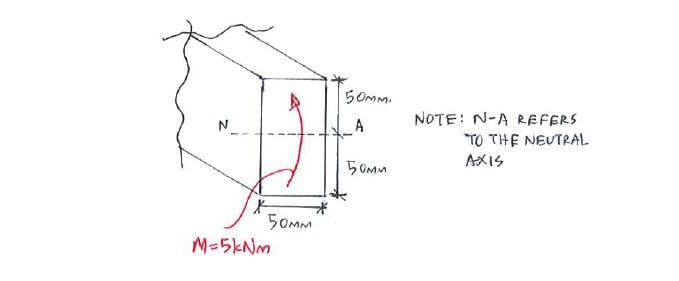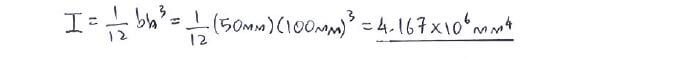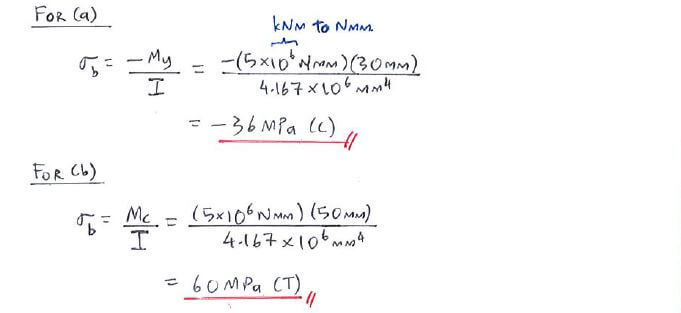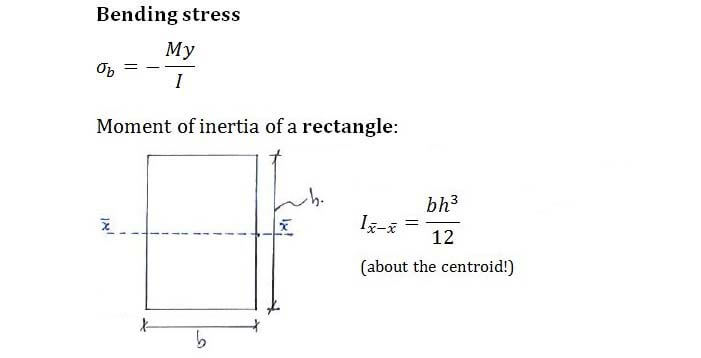# Example QuestionClick below to show answer. X

#### Step 1: Calculate moment of inertia I Step 1: Calculate moment of inertia I#### Step 2: Calculate bending stress σb Step 2: Calculate bending stress σb#### Useful formula: Useful formula:Now that you've mastered this question, you can try Question 1.